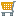You are viewing the RapidMiner Studio documentation for version 9.6 - Check here for latest version

#Create Association Rules (RapidMiner Studio Core)

## Synopsis

This operator generates a set of association rules from the given set of frequent itemsets.

## Description

Association rules are if/then statements that help uncover relationships between seemingly unrelated data. An example of an association rule would be "If a customer buys eggs, he is 80% likely to also purchase milk." An association rule has two parts, an antecedent (if) and a consequent (then). An antecedent is an item (or itemset) found in the data. A consequent is an item (or itemset) that is found in combination with the antecedent.

Association rules are created by analyzing data for frequent if/then patterns and using the criteria support and confidence to identify the most important relationships. Support is an indication of how frequently the items appear in the database. Confidence indicates the number of times the if/then statements have been found to be true. The frequent if/then patterns are mined using the operators like the FP-Growth operator. The Create Association Rules operator takes these frequent itemsets and generates association rules.

Such information can be used as the basis for decisions about marketing activities such as, e.g., promotional pricing or product placements. In addition to the above example from market basket analysis association rules are employed today in many application areas including Web usage mining, intrusion detection and bioinformatics.

## Input

•item sets (Frequent Item Sets)

This input port expects frequent itemsets. Operators like the FP-Growth operator can be used for providing these frequent itemsets.

## Output

•item sets (Frequent Item Sets)

The itemsets that was given as input is passed without changing to the output through this port. This is usually used to reuse the same itemsets in further operators or to view the itemsets in the Results Workspace.

•rules (Association Rules)

The association rules are delivered through this output port.

## Parameters

• criterionThis parameter specifies the criterion which is used for the selection of rules.
• confidence: The confidence of a rule is defined conf(X implies Y) = supp(X ∪Y)/supp(X) . Be careful when reading the expression: here supp(X∪Y) means "support for occurrences of transactions where X and Y both appear", not "support for occurrences of transactions where either X or Y appears". Confidence ranges from 0 to 1. Confidence is an estimate of Pr(Y | X), the probability of observing Y given X. The support supp(X) of an itemset X is defined as the proportion of transactions in the data set which contain the itemset.
• lift: The lift of a rule is defined as lift(X implies Y) = supp(X ∪ Y)/((supp(Y) x supp(X)) or the ratio of the observed support to that expected if X and Y were independent. Lift can also be defined as lift(X implies Y) =conf(X implies Y)/supp(Y). Lift measures how far from independence are X and Y. It ranges within 0 to positive infinity. Values close to 1 imply that X and Y are independent and the rule is not interesting.
• conviction: conviction is sensitive to rule direction i.e. conv(X implies Y) is not same as conv(Y implies X). Conviction is somewhat inspired in the logical definition of implication and attempts to measure the degree of implication of a rule. Conviction is defined as conv(X implies Y) =(1 - supp(Y))/(1 - conf(X implies Y))
• gain: When this option is selected, the gain is calculated using the gain theta parameter.
• laplace: When this option is selected, the Laplace is calculated using the laplace k parameter.
• ps: When this option is selected, the ps criteria is used for rule selection.
Range: selection
• min_confidenceThis parameter specifies the minimum confidence of the rules. Range: real
• min_criterion_valueThis parameter specifies the minimum value of the rules for the selected criterion. Range: real
• gain_thetaThis parameter specifies the parameter Theta which is used in the Gain calculation. Range: real
• laplace_kThis parameter specifies the parameter k which is used in the Laplace function calculation. Range: real

## Tutorial Processes

### Introduction to the Create Association Rules operator

The 'Iris' data set is loaded using the Retrieve operator. A breakpoint is inserted here so that you can view the ExampleSet. As you can see, the ExampleSet has real attributes. Thus the FP-Growth operator cannot be applied on it directly because the FP-Growth operator requires all attributes to be binominal. We have to do some preprocessing to mold the ExampleSet into desired form. The Discretize by Frequency operator is applied to change the real attributes to nominal attributes. Then the Nominal to Binominal operator is applied to change these nominal attributes to binominal attributes. Finally, the FP-Growth operator is applied to generate frequent itemsets. The frequent itemsets generated from the FP-Growth operator are provided to the Create Association Rules operator. The resultant association rules can be viewed in the Results Workspace. Run this process with different values for different parameters to get a better understanding of this operator.